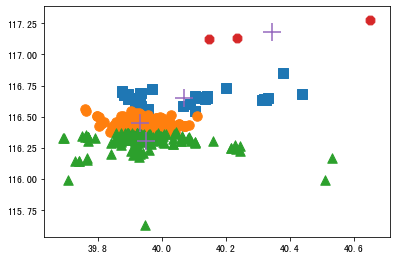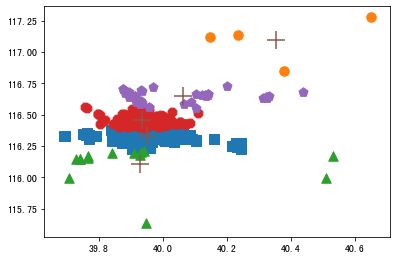# Lab7 k-Means应用实践

## 实验环境

### 硬件

• Intel(R) Core(TM) i7-6567U CPU @3.30GHZ 3.31GHz
• 8.00GB RAM

### 软件

• Windows 10, 64-bit (Build 17763) 10.0.17763
• Visual Studio Code 1.39.2
• Python 2019.10.41019：九月底发布的 VSCode Python 插件支持在编辑器窗口内原生运行 juyter nootbook 了，非常赞！
• Remote - WSL 0.39.9：配合 WSL，在 Windows 上获得 Linux 接近原生环境的体验。
• Windows Subsystem for Linux [Ubuntu 18.04.2 LTS]：WSL 是以软件的形式运行在 Windows 下的 Linux 子系统，是近些年微软推出来的新工具，可以在 Windows 系统上原生运行 Linux。
• Python 3.7.4 64-bit (‘anaconda3’:virtualenv)：安装在 WSL 中。

## 获取地图数据

### 使用 API 获取数据

``````http://api.map.baidu.com/place/v2/search?q=查询内容&page_size=范围记录数量&page_num=分页页码&region=地区&output=数据格式&ak=秘钥
``````

## kMean 算法实现

### 建立辅助函数函数

`loadDataSet()`读取文件的数据，函数`distEclud()`计算两个向量的欧式距离，函数`randCent()`随机生成 k 个随机质心。

``````>>> import kmeans
>>> from numpy import*
#从文本文件中构建矩阵：
#测试函数randCent()：
>>>min(dataset[:,0])
>>>min(dataset[:,1])
>>>max(dataset[:,1])
>>>max(dataset[:,0])
#查看randCent()能否生成min到max之间的值：
>>>kmeans.randCent(dataset, 2)
#测试距离计算函数：
>>>kmeans.distEclud(dataset, dataset)
``````

### kMean 算法实现

``````>>>dataset=mat(kmeans.loadDataSet('testSet.txt'))
#查看聚类结果：
>>>myCentroids,clustAssing=kmeans.kMeans(dataset,4)
#参看迭代的次数及结果：>>>myCentroids2
>>>clustAssing
``````

## 用 kMeans 算法对地图上的点聚类

### 准备数据

``````>>>dataMat=loadDataSet('Restaurant_Data_Beijing.txt')
>>>dataMat
``````

### 对地理坐标进行聚类

``````>>>kmeans.clusterPlaces
``````

``````>>>kmeans.clusterPlaces(4)
>>>kmeans.clusterPlaces(5)
>>>kmeans.clusterPlaces(6)
``````

## 操作习题

``````def geoGrab():

import json
import urllib.request

j = 0
f = open(r'Restaurant_Data_Beijing.txt', 'w')

for j in range(0, 20):

b = '&region=%E5%8C%97%E4%BA%AC&output=json&ak=Oz3v1GzmrxmtYpiEmaZaxhrWo3YS5NNr'

# 上面的汉字(百分号部分)做了urlencode处理，原本是」饭店」和」北京」
# 密钥需要自己申请，然后替换掉上面的「秘钥」
c = str(j)
url = a+c+b
j = j+1

# '&page_num=19&region=%E5%8C%97%E4%BA%AC&output=json&ak=qUPyb0ZPGmT41cL9L5irQzcnc48yIEck'
temp = urllib.request.urlopen(url)

# 把字符串解析成为Python对象
i = 0

for i in range(0, 20):

lat = hjson['results'][i]['location']['lat']
lng = hjson['results'][i]['location']['lng']
print('%s\t%f\t' % (lat, lng))
f.write('%s\t%f\t\n' % (lat, lng))
i = i+1
f.close()

geoGrab()
``````

### kmeans.py 中的语句「from numpy import* 」用语句「import numpy as np」代替，修改其中对应的代码，使其能够正常执行

``````# -*-coding:utf-8-*-
import matplotlib
import matplotlib.pyplot as plt
import numpy as np

dataMat = []
fr = open(fileName)

curLine = line.strip().split('\t')

# 将所有数据转换为float类型
fltLine = list(map(float, curLine))
dataMat.append(fltLine)
return dataMat

def distEclud(vecA, vecB):

return np.sqrt(sum(np.power(vecA - vecB, 2)))

def randCent(dataSet, k):

# 得到数据集的列数
n = np.shape(dataSet)

# 得到一个K*N的空矩阵
centroids = np.mat(np.zeros((k, n)))

# 对于每一列
for j in range(n):

# 得到最小值
minJ = min(dataSet[:, j])

# 得到当前列的范围
rangeJ = float(max(dataSet[:, j]) - minJ)

# 在最小值和最大值之间取值
centroids[:, j] = np.mat(minJ + rangeJ * np.random.rand(k, 1))
return centroids

def distSLC(vecA, vecB):

# pi为圆周率，在导入numpy时就会导入的了
# sin(),cos()函数输出的是弧度为单位的数据
# 由于输入的经纬度是以角度为单位的，故要将其除以180再乘以pi转换为弧度
# 设所求点A ，纬度β1 ，经度α1 ；点B ，纬度β2 ，经度α2。则距离
# 距离 S=R·arc cos[cosβ1cosβ2cos（α1-α2）+sinβ1sinβ2]

a = np.sin(vecA[0, 1]*np.pi/180) * np.sin(vecB[0, 1]*np.pi/180)
b = np.cos(vecA[0, 1]*np.pi/180) * np.cos(vecB[0, 1]*np.pi/180) * \
np.cos(np.pi * (vecB[0, 0]-vecA[0, 0]) / 180)

return np.arccos(a + b)*6371.0  # 6371.0为地球半径

def kMeans(dataSet, k, distMeas=distEclud, createCent=randCent):

# 数据集的行数，即数据的个数
m = np.shape(dataSet)

# 簇分配结果矩阵
clusterAssment = np.mat(np.zeros((m, 2)))

# 第一列储存簇索引值
# 第二列储存数据与对应质心的误差
# 先随机生成k个随机质心的集合
centroids = createCent(dataSet, k)
clusterChanged = True

# 当任意一个点的簇分配结果改变时
while clusterChanged:
clusterChanged = False

# 对数据集中的每一个数据
for i in range(m):
minDist = np.inf
minIndex = -1

# 对于每一质心
for j in range(k):

# 得到数据与质心间的距离
distJI = distMeas(centroids[j, :], dataSet[i, :])

# 更新最小值
if distJI < minDist:
minDist = distJI
minIndex = j

# 若该点的簇分配结果改变
if clusterAssment[i, 0] != minIndex:
clusterChanged = True
clusterAssment[i, :] = minIndex, minDist**2

# print centroids
# 对于每一个簇
for cent in range(k):

# 通过数组过滤得到簇中所有数据
ptsInClust = dataSet[np.nonzero(clusterAssment[:, 0].A == cent)]

# .A 方法将matrix类型元素转化为array类型
# 将质心更新为簇中所有数据的均值

centroids[cent, :] = np.mean(ptsInClust, axis=0)
# axis=0表示沿矩阵的列方向计算均值
return centroids, clusterAssment

def clusterPlaces(numClust=5):

datList = []

lineArr = line.split('\t')
datList.append([float(lineArr), float(lineArr)])
datMat = np.mat(datList)

# 进行聚类
myCentroids, clustAssing = kMeans(datMat, numClust, distMeas=distSLC)
fig = plt.figure()

# 创建一个矩形
rect = [0.1, 0.1, 0.8, 0.8]

# 用来标识簇的标记
scatterMarkers = ['s', 'o', '^', '8', 'p',
'd', 'v', 'h', '>', '<']
axprops = dict(xticks=[], yticks=[])

for i in range(numClust):

ptsInCurrCluster = datMat[np.nonzero(clustAssing[:, 0].A == i), :]
markerStyle = scatterMarkers[i % len(scatterMarkers)]
ax1.scatter(ptsInCurrCluster[:, 0].flatten(
).A, ptsInCurrCluster[:, 1].flatten().A, marker=markerStyle, s=90)
ax1.scatter(myCentroids[:, 0].flatten().A,
myCentroids[:, 1].flatten().A, marker='+', s=300)

plt.show()

if __name__ == '__main__':
clusterPlaces(6)
``````

### 对聚类结果可视化（包含样本点和蔟中心，用不同颜色、记号标记）

``````for i in range(4,7):
clusterPlaces(i)
``````